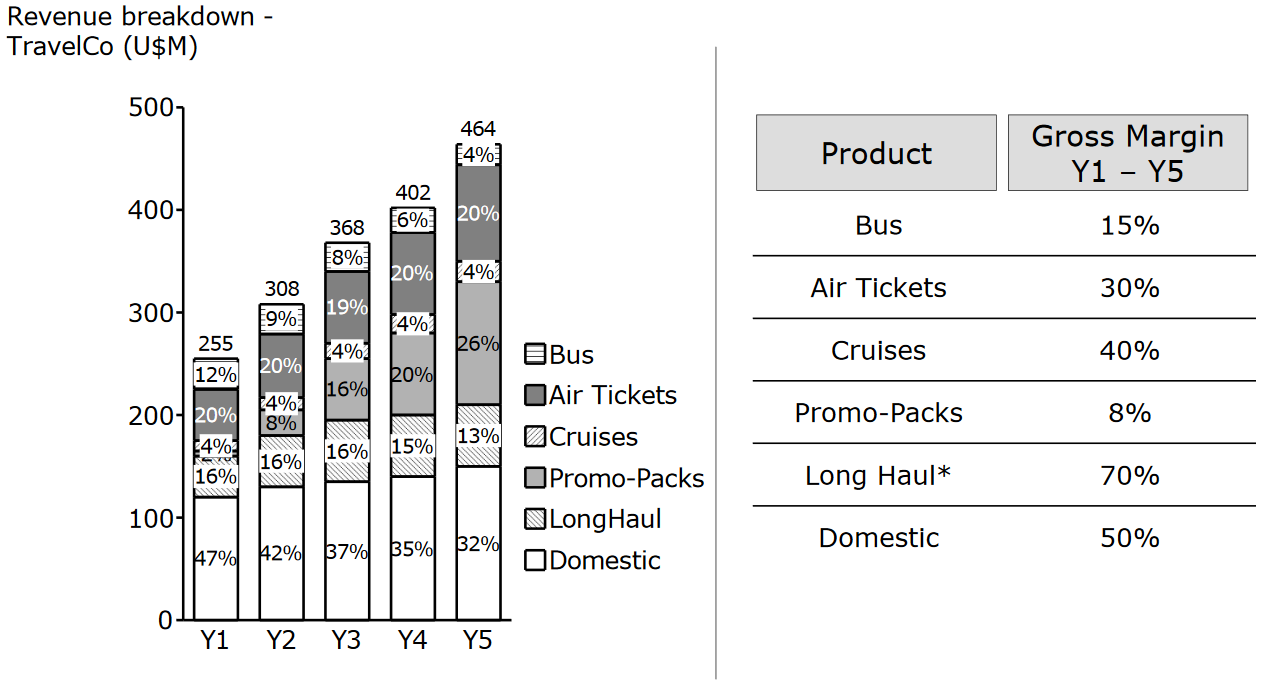# Calculate gross margin using revenue breakdown

Recent activity on Mar 24, 2019
3.0 k Views

Hello! I'm doing a sample case and I'd like to know how can I find what year had the highest gross margin from a chart.

I know the gross margin would be the revenue minus the cost but I know there would be a more simple way since I do not have the cost in this chart but have the revenue breakdown year by year.

This looks like a simple question but I am not 100% sure how I can solve this.Thanks in advance for the help!

• Date ascending
• Date descending

Y1: 0.47x0.5 + 0.16x0.7 + 0.02x0.08 + 0.04x0.4 + 0.2x0.3 + 0.12x0.15 = 0.4426

Y2: 0.42x0.5 + 0.16x0.7 + 0.08x0.08 + 0.04x0.4 + 0.2x0.3 + 0.09x0.15 = 0.4179

Y3: 0.37x0.5 + 0.16x0.7 + 0.16x0.08 + 0.04x0.4 + 0.19x0.3 + 0.08x0.15 = 0.394

Y4: 0.35x0.5 + 0.15x0.7 + 0.2x0.08 + 0.04x0.4 + 0.2x0.3 + 0.06x0.15 = 0.381

Y5: 0.32x0.5 + 0.13x0.7 + 0.26x0.08 + 0.04x0.4 + 0.2x0.3 + 0.04x0.15 = 0.3538

Y1 has the highest gross margin of all years

I think you can calculate the gross margin of each year as a weighted average of the gross margin of each of the segments, with the contribution of that segment to the total revenue as the weighing factor. So for each of the years, the overall gross margin would be contribution (bus) * gross margin (bus) + contribution (air tickets) * gross margin (air tickets) and so on, divided by the number of contributions.

To speed things up, you can use the fact that you won't need absolute value to sort them. You can therefore skip the division step, as the number of contributions is the same for each year (6) and that factor effectively cancels out. You can also remove some contributions that are sufficiently small or that are similar and therefore should cancel out - for example, air tickets contribute approximately 20% to the revenue in each of the years, and you will therefore arrive at the same order if you neglect this contribution.